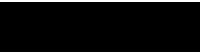# Math Lessons for ESL Students: Learning Plus and Minus

## Add Plus MoreBegin by having students count up to twenty. Have each student count one number until, as a group, they reach twenty. Then write 1+1 on the board and ask them to tell you what it says, in English. Some students may already know that you can say, "One plus one equals two." If no one knows this, then you can be sure that all this information is new, and you can slow down.

Write on the board:

Add = increasing numbers, combining numbers to make a bigger number.

Plus = (+) a math symbol that means "more"

• Have everyone stand up and begin calling students to the front of the class by saying, "One student (say student's name) PLUS another student (say student's name) EQUALS how many students?" Have the class answer this question and write down the equation as words and as numbers.

(One students plus one student equals two students) and (1+1=2)

• Do this for a variety of equations. Then have students write five of their own questions. When they finish, have them work in pairs of two asking and answering with their classmate.
• If there is extra time, have them draw a beautiful plus sign with the word "PLUS" written elegantly above it. This will help them remember that + is called "plus" in English. To help organize your lesson, you may consider an ESL lesson plan template.

## Subtract, Take Away, and Minus.Have all of your students stand up again, but this time write the number of students standing. For this example let's say you have 20 students in the class. Then say, "20 minus 2 equals…?" and motion for two students to sit down. Continue this process until there are no more students standing. Then repeat the process with the words "take away", instead of "minus" to show that they are synonyms.

Write on the board:

Subtract = decreasing numbers, making smaller numbers

Minus / Take Away = (-) a math symbol that means "less"

Write 20 math equations for plus and twenty for minus. Give each student a couple of cards and have them ask their partner to answer the question by listening.

Example: Student A is holding a card that says, "6+2=___?" ( On the back it says "answer 8"). Student A says, "What does six plus two equals?" Student B Answers, "Six plus two equals eight." And then this group switches cards with another group and student B asks the question.

Also, if their is additional time, feel free to have students draw up some math art, with a (-) minus theme, that they can share with the class later.

## Homework

Give students word problems that include the written numbers.

What does seven plus two equal?

What is fifteen minus nine?

What is nine take away four?

What is thirteen plus three?

Images

Wikimedia Commons – Plus

Wikimedia Commons – Minus

## This post is part of the series: ESL Lesson Plans

More isn’t always better, unless you are talking about ESL lesson plans.